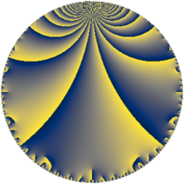# Properties

 Label 225.6.hLevel $225$ Weight $6$ Character orbit 225.h Rep. character $\chi_{225}(46,\cdot)$ Character field $\Q(\zeta_{5})$ Dimension $244$ Sturm bound $180$

# Learn more about

## Defining parameters

 Level: $$N$$ $$=$$ $$225 = 3^{2} \cdot 5^{2}$$ Weight: $$k$$ $$=$$ $$6$$ Character orbit: $$[\chi]$$ $$=$$ 225.h (of order $$5$$ and degree $$4$$) Character conductor: $$\operatorname{cond}(\chi)$$ $$=$$ $$25$$ Character field: $$\Q(\zeta_{5})$$ Sturm bound: $$180$$

## Dimensions

The following table gives the dimensions of various subspaces of $$M_{6}(225, [\chi])$$.

Total New Old
Modular forms 616 252 364
Cusp forms 584 244 340
Eisenstein series 32 8 24

## Trace form

 $$244q - q^{2} - 947q^{4} - 109q^{5} - 50q^{7} - 622q^{8} + O(q^{10})$$ $$244q - q^{2} - 947q^{4} - 109q^{5} - 50q^{7} - 622q^{8} - 119q^{10} - 244q^{11} - 543q^{13} - 2059q^{14} - 17847q^{16} + 1792q^{17} - 1193q^{19} + 3529q^{20} + 4696q^{22} + 3335q^{23} - 14171q^{25} + 14454q^{26} - 24005q^{28} + 4159q^{29} + 11295q^{31} + 16830q^{32} + 24705q^{34} + 4585q^{35} - 7615q^{37} - 12479q^{38} + 80102q^{40} - 21332q^{41} + 39066q^{43} - 16062q^{44} + 2497q^{46} - 58282q^{47} + 516086q^{49} + 20959q^{50} - 186972q^{52} + 41805q^{53} - 16456q^{55} + 68730q^{56} + 251198q^{58} + 49788q^{59} + 22705q^{61} - 261270q^{62} - 414852q^{64} - 230232q^{65} + 71038q^{67} + 123898q^{68} + 72240q^{70} + 44884q^{71} + 49185q^{73} + 86376q^{74} - 61336q^{76} + 347620q^{77} + 28191q^{79} + 459204q^{80} + 540890q^{82} - 305823q^{83} - 9362q^{85} - 221259q^{86} - 54568q^{88} + 123932q^{89} + 182472q^{91} + 153810q^{92} + 21313q^{94} + 44069q^{95} + 390218q^{97} - 315128q^{98} + O(q^{100})$$

## Decomposition of $$S_{6}^{\mathrm{new}}(225, [\chi])$$ into newform subspaces

The newforms in this space have not yet been added to the LMFDB.

## Decomposition of $$S_{6}^{\mathrm{old}}(225, [\chi])$$ into lower level spaces

$$S_{6}^{\mathrm{old}}(225, [\chi]) \cong$$ $$S_{6}^{\mathrm{new}}(25, [\chi])$$$$^{\oplus 3}$$$$\oplus$$$$S_{6}^{\mathrm{new}}(75, [\chi])$$$$^{\oplus 2}$$